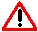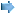# Creating scripts for Iris

First, consult Christian Buil webpage dedicated to scripts. Analyse the scripts proposed by Skyimaging and try to understand the code. In case of doubt, consult Iris command list.

Remark 1

All scripts must have the extension .pgm

Example: script.pgm

Remark 2

Instructions written in scripts are the same ones you write in the command box, but without the > sign in front. For exemple, type as follows to load 3 images and save them under a different name.

>load image1
>save photo1
>load image2
>save photo2
>load image3
>save photo3

Remark 3

A script contains a maximum of 5 parameters, indicated by a \$ sign.

Remark 4

It is useful to comment your scripts so that you can modify them, even a few years later. All characters that Iris does not recognize as an instruction are classed as comments. In my scripts, I use lines of ********** to separate different processing steps. I recommend you do not use other character types to avoid crashing Iris (it happened to me once with lines of ----------- or _____________)

To know at all times where you are while running a script, use the « echo » command, which displays a message in the IRIS output window. If you need to have a manual command in a script, type in the .pgm file what you need to input in that step under a echo command and have the script pause with the use on the prompt command.

Remark 5

Iris sometimes need the user to select an area within an image. You can do it manually by pausing the script (« prompt » command) but you then need to have a manual input. Alternatively, you can use the mouse_select command which automatically selects an area within an image. Therefore > mouse_select 1 1 640 480 selects automatically the useful area (the planet) by looking in the whole image (in this example, a 640 x 480 pixel image), which unfortunately slows down some command execution, such as registration (unlike the use of prompt windows). The use of mouse_select is explained here.

Remark 6

Processing a planet movie is done following various steps. Some steps must be done in a particular order. To follow this order, refer to skyimaging and to Christian Buil tutorials.

For example, I try to follow these principles:

• Normalise the background (noffset) before selecting and registering the images, etc
• If you need to compensate for field rotation, do it at the start of the process. If there are dust spots on the sensor, use a flat field first before rotating the images.
• Select the images using bestof then register, not the other way round. Thats what you learn in the Christian Buil tutorials.
• ...

Remark 7

Certain commands require that you load a reference image before running that command. Do not forget!

Remark 8

Be patient when writing a script. Be rigourous and test it first on a short section of your video. When you are satisfied it works well, apply it to the whole video. The first scripts are the hardest to write. The later ones are done faster as you have a better understanding of Iris. You can also then use various parts of different scripts to create new ones.

Remark 9

If you have a movie captured with a color webcam, you can process the three colors at once by using T-TOOLS commands. I believe that these commands should only be used in scripts. Using them manually does not bring any advantage (you cannot go back if it goes wrong), thats why I used simple commands with different input and output names in my various tutorials. T-TOOLS are very easy to use: every time you see in a tutorial a command that must be applied to each color channel, look into the T-TOOLS command list for a command that does all three commands at once.

For example, instead of a « pregister » on the green channel, followed by two « file_trans » to the red and blue channels, you can simply use « t_register » found in T-TOOLS.T_tools requires your starting sequences to be named r, g, and b!

Here is the list of T_TOOLS commands uploaded from Christian Buil website. Those marked with an arroware the most important in my opinion.

T_ADD [R] [G] [B] [NUMBER]

Addition of the images of each color planes (equivalent ADD2). Operation carried out:

(R1+R2+..+Rn, G1+G2+..+Gn, B1+B2+..+Bn) -> [R], [G], [B]T_ADD_NORM [R] [G] [B] [NUMBER]

Even operation that T_ADD but standardizes the images on level 32000 with the need if this level is exceeded (equivalent ADD_NORM).

T_COMPOSIT [R] [G] [B] [SIGMA] [NB ITER] [FLAG MAX] [NUMBER]

Produce three images [R] [G] and [B] by making an optimal addition of the images of the sequences R1... Rn, G1... Gn, B1... Bn (equivalent COMPOSIT). The procedure is iterative if [NB ITER] is higher than 1. The threshold of rejection of the bad values is adjusted by the variable [SIGMA] (typical real value between 2 and 3). Level 32000 after addition is not exceeded if FLAG MAX = 1 (a normalization of the intensities is done). Limited to 19 input frames.

T_COPY [in R] [in G] [in B] [out R] [out G] [out B] [NUMBER]

Duplicate a trichromatic sequence. Carry out the operation:

(in R1... in Rn, G1... in Gn, B1... in Bn) -> (out R1... out Rn, out G1... out Gn, out B1... out Bn)T_CREGISTER [THRESHOLD] [NUMBER]

Registration of planetary images by adjusting a circle on level [THRESHOLD] around planet limb (equivalent to CREGISTER).

T_DIV [R] [G] [B] [NUMBER]

Divide the sequences R1... Rn, G1... Gn, B1... Bn by the images [R], [G] and [B] (equivalent has DIV, but the parameters of standardization is calculated automatically in T_DIV). Carry out the operation:

(R1/[R]... Rn/[R], G1/[G]... Gn/[G], B1/[b]... Bn/[B]) -> (R1... Rn, G1... Gn, B1... Bn)

T_GAUSS [SIGMA]

Convolution by Gaussian of the trichromatic image defined by the file R, B and B. Equivalent to command GAUSS2.

T_MULT [coef R] [coef G] [coef B] [NUMBER]

Multiply each image of the sequences R1... Rn, G1... Gn, B1... Bn by constants (equivalent with MULT2). Carry out the operation:

(R1*[coef R]... Rn*[coef R], G1*[coef G]... Gn*[coef G], B1*[coef B]... Bn*[coef B]) - > (R1... RN, G1... Gn, B1... Bn)

T_NGAIN [NORM] [NUMBER]

Multiply each images of the 3 sequences by a constant calculated by Iris in manner what the median level of each image is equal to [NORM] (equivalent with NGAIN2). This command is in particular used for stack flat-field images before median composite.T_NOFFSET [NORM] [NUMBER]

Add (or subtract) to each images of the three sequences a constant calculated by Iris in manner what the median level of each image is equal to [STANDARD] (equivalent NOFFSET2). Useful to bring at the same the level the sky background on deep-sky images.

T_OFFSET [offset R] [offset G] [offset B] [NUMBER]

Add a constant to each images of the sequences R1... Rn, G1... Gn, B1... Bn (equivalent with #OFFSET2). The constants can have negative values. Carry out the operation:

(R1+[offset R]... +.Rn+[offset R], G1+[offset G]+... +Gn+[offset G], B1+[offset B]+... +Bn+[offset B]) - > (R1... RN, G1... Gn, B1... Bn)T_PREGISTER [SIZE] [NUMBER]

Equivalent with PREGISTER for the registration of the planetary images. Registration is calculated on the sequence G1... Gn then is the parameters of translation are applied to the sequences R1... Rn, B1... Bn. It is thus supposed that it is the green component of the three-colour process which presents the best details and contrasts.

T_PREREGISTER [NUMBER]

Equivalent with command PREREGISTER.

T_REGISTER [NUMBER]

Equivalent with command REGISTER. Ideal for the deep-sky images (uses the position of a reference star to be selected in the first images of one of the series, image G1.FIT for example).

T_RESTORE

Carry out the opposite operation of T_STORE : restore in the files image R, G and B the contents of file # R, # G and # B.

T_SCALE [OPTION] [FX] [FY]

Change the scale of a trichromatic image defined by the files R, G and B. Same parameters as command SCALE.T_SELECT

Simultaneous sort by decreasing quality the images in the red, green and blue planes (equivalent to SELECT). It is necessary to have run before command BESTOF on one of the components trichromatic (the green one for example).

T_SMEDIAN [R] [G] [B] [NUMBER]

Median stack of the sequences R1... Rn, G1... Gn, B1... Bn by producing the images [R] [G] and [B] (equivalent with SMEDIAN). Algorithm fast but limited to 19 images.

T_SMEDIAN2 [R] [G] [B] [NUMBER]

Even function that T_SMEDIAN, slightly slower but the number of images is unlimited (equivalent to SMEDIAN2).

T_STORE

Copy the three images having for name R, G and B respectively in files # R, # G and # B.

T_SUB [R] [G] [B] [NUMBER]

Subtract the images [R], [G] and [B] to the sequences R1... Rn, G1... Gn, B1... Bn (equivalent with SUB2 or SUB). Carry out the operation:

(R1-[R]... Rn-[R], G1+[G]... Gn+[G], B1+[B]... Bn+[B]) - > (R1... Rn, G1... Gn, B1... Bn)

T_TRICHRO or T_TR

Display a color image color from the files images R, G and B.

T_UNSHARP [SIGMA] [COEF] [FLAG]

Display a color image starting from the files images R, G and B, but applies a unsharp masking filter with each components as a preliminary. Same parameters as command UNSHARP.

T_UNSHARP2 [SIGMA] [COEF] [FLAG] [NUMBER]

Calculate the unsharp masking of each images of the three sequences (equivalent with UNSHARP2).

Have fun!

© 2021 Leboutte Sebastien - http://www.skyimaging.com -